Although the conoid term has different meanings according to the context in which it is used, in the field of tensile architecture, we use it to refer to those forms of revolution, similar to a cone, where the curve is not a straight line but a concave curve as seen from outside.

If the conoid is perfectly regular, the base would be a circle (as happens in cone). While it is possible to use perfectly regular conoids, the vast majority of them have regular polygonal base, i.e., the perimeter of the base has pronounced vertices.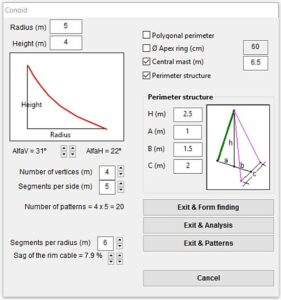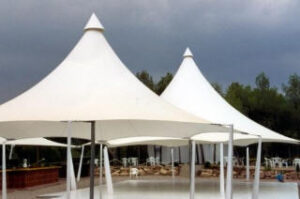WinTess3 allows you to quickly generate this type of regular polygonal conoids through the menu File | New | Conoid. Conoid generator window needs certain information to create the object:

The radius of the circle confined at the base of the membrane.
• Height
Height of the conoid itself. That is to say, from the base of the membrane to the top. The height of the support elements are not counted, i.e. masts, tripods etc.
• Generatrix
Under the radius and height values is a box where the generatrix is drawn in red. To modify this generatrix, we have two plus/minus controls. The left control causes more sudden changes, while the right one causes more smooth. We know the numerical values of the generatrix through data AlfaV which is the angle between the vertical and the tangent of the curve at the top, and AlfaH which is the angle between the horizontal with the tangent of the curve at the base.
• Number of vertices
Regarding the base polygon of the conoide.
• Segments per side
This value is used by the program to generate the mesh and, more importantly, the patterns of the membrane. Each segment will be a pattern. Therefore in a conoid with 4 vertices using 5 segments per side, we will need a total of 20 patterns of fabric to make the conoid.
This setting will provide a tighter main curvature. In general we use values between 5 and 8. If we use less curvature, the conoid will be modest and planar, while if we use more we will not gain great precision and design process will clearly slow down.
• Sag of the rim cable
With this percent value, we adjust the curvature of the boltrope cable that form the perimeter of the conoid base. The entry of this value is not numeric, but it is done through two plus/minus controls. The one on the left leads to more drastic changes, while the one on the right the causes more soft.

Once we have found the generator that satisfies our design, we can move on to consider the general controls on the right-hand side of the window:

• Polygonal perimeter
If we mark this option, the conoid base will be a perfect polygon, i.e. the boltrope from vertex to vertex will be straight.
• Apex ring
We can trim the membrane at the apex of the conoid forming a circle of diameter that we set in the corresponding box. You have to be careful in the height of the conoid, since it takes into account the height of the membrane (apex ring) not the theoretical point of the apex.
If the user checks Apex Ring check box, automatically this window expands to the right side and we get a selector for the ring support structure: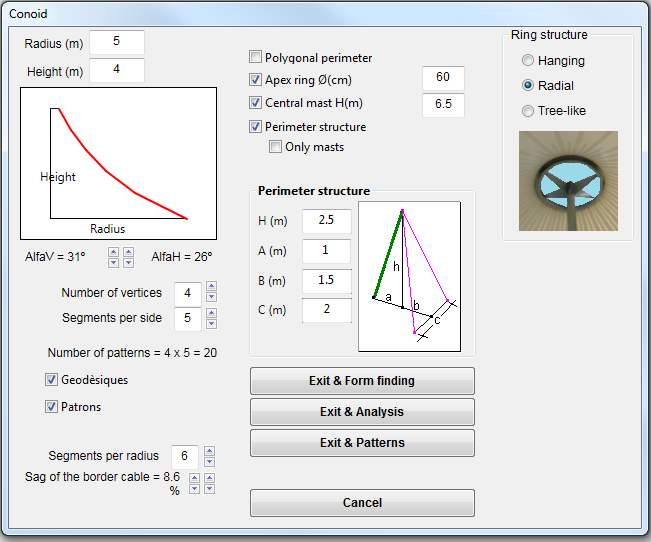1. Hanging: The ring is hanging from the highest tip of the central mast by means of some cables.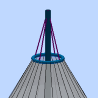2. Radial: The ring is supported by means of some built-in to the central mast horizontal radii.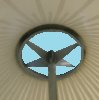3. Tree-like: The ring is supported by some tubes like branches, upwards from the top of the central mast.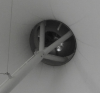• Central mast
We can decide if we want a central mast or not. In case it exists, it is necessary to define its height. Typically will be higher than the conoid protruding below. But there is no such an obligation.
• Perimeter structure
We may decide to include a support system at the perimeter vertices of the conoid. These supports are defined by 4 values:
• Height (H)
It is the height of the vertex with respect to the ground. That is why it is not equal to the length of the perimeter masts or its guyropes. If this value is set to zero, it means there is no structure at the perimeter.
• Inclination of the perimeter mast (A)
Value that measures the inclination of the mast perimeter as the distance between the its base and the projection of the vertex on the floor. It can be zero (vertical mast), positive (mast tilted outward) or negative (mast tilted inward).
• Inclination of guyropes (B)
Value that measures the inclination of the guyropes of perimeter mast  as the distance between the midpoint of the base of these guyropes and the projection the vertex on the floor. It can be zero (vertical guyrope if there is only one) and positive (outward guyropes) or negative (inward guyropes).
• Distance between guyropes (C)
Usually, two guyropes are used by each perimeter mast as the tripod is much more stable. The distance between these two guyropes is measured by the distance between the respective bases. If this value is zero, it means that there is only one guyrope. A negative value doesn’t make any sense.

As we go by modifying any of the above parameters, we see that the model drawn in the main window adapts to these changes. When we finally reached the desired model, we can leave this window through three buttons (three possibilities):

• Exit & Form finding
Through this button, we return to the main window of WinTess3, in the state of Form finding. We use this possibility when we want get a irregular conoid from completely regular one. We can modify the position of the apex, modify any vertex, etc.
• Exit & Analysis
Through this button, we return to the main window of WinTess3, in the state of Analysis. We use this possibility when the final form has been achieved and we wish to do calculation of the conoid under the loads of snow, wind, etc.
• Exit & Patterning
Through this button we return to the main window of WinTess3, in the state of Patterning. We use this possibility when the final form has been achieved and we want perform its patterning. It is assumed that the calculation is not relevant, either because the actions are very small or because we have done it previously.